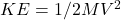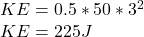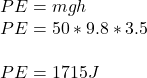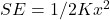## At the top of a giant swing on the high bar, a 50 kg gymnast’s velocity is 3 m/s, and he is 3.5 m high. What is the gymnast’s total mechanic

Question

At the top of a giant swing on the high bar, a 50 kg gymnast’s velocity is 3 m/s, and he is 3.5 m high. What is the gymnast’s total mechanical energy (KE + PE+ SE) at this instant?

in progress 0
2 weeks 2021-08-30T20:50:55+00:00 1 Answers 0 views 0

Total Mechanical Energy of the gymnast = 1940J = 1.94 kJ

Explanation:

Total Mechanical Energy = KE + PE + SE

Kinetic Energy,Potential Energy,Strain Energy,Since there is no force of deformation, x = 0, SE = 0

Total Mechanical Energy = 225 + 1715

Total Mechanical Energy of the gymnast = 1940J = 1.94 kJ